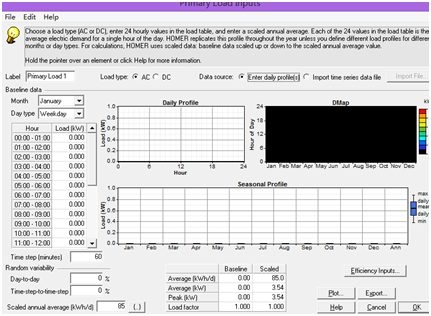## 2015年2月16日星期一

### MATLAB simulation

our group optimized our design of micro power system regards on the Diavik Diamond Mine, which is Canada's largest diamond mine. I was responsible for estimating the power in load.Figure 1 shows the primary load of Power Energy. The load requires the specific power
consumption. However, we only has the data of the total power consumption of a year in this case. Due to the limitation of the data, Mento-Carlo medal was applied to simulate the power consumption in each month.

Figure 2. Results of MATLAB simulationFigure 4. Distribution of simulated power
According to the data, Diavik Diamond Mine consumes 16977 MWH power each year, with peak power in winter. Figure 2 to figure 4 shows the results that simulated by MATLAB. Mento-Carlo method is applied to generate random data. According to the data that the peak power in a city is two times the low demand period.